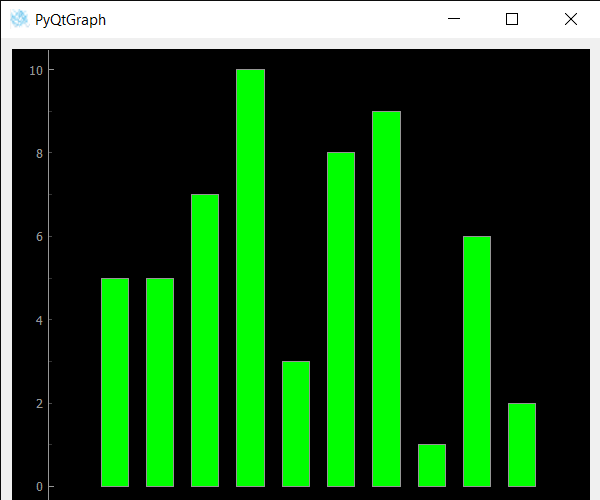Skip to content
Related Articles
PyQtGraph – View Ranged Changed Event for Bar Graph
• Last Updated : 14 Oct, 2020

In this article we will see how we can create a view range changed event with the bar graph in the PyQtGraph module. PyQtGraph is a graphics and user interface library for Python that provides functionality commonly required in designing and science applications. Its primary goals are to provide fast, interactive graphics for displaying data (plots, video, etc.) and second is to provide tools to aid in rapid application development (for example, property trees such as used in Qt Designer).A bar chart or bar graph is a chart or graph that presents categorical data with rectangular bars with heights or lengths proportional to the values that they represent. The bars can be plotted vertically or horizontally. A vertical bar chart is sometimes called a column chart. This event get triggered when the view ranged of the bar graph is changed. When we move the graph above the view range this event get triggered

We can create a plot window and bar graph with the help of commands given below

```# creating a pyqtgraph plot window
window = pg.plot()

# creating a bar graph of green color
bargraph = pg.BarGraphItem(x=x, height=y1, width=0.6, brush='g')
```

In order to do this we have modify the bar graph class, below is the class which can be used

```# Bar Graph class
class BarGraphItem(pg.BarGraphItem):

# constructor which inherit original
# BarGraphItem
def __init__(self, *args, **kwargs):
pg.BarGraphItem.__init__(self, *args, **kwargs)

# creating view range changed event
def viewRangeChanged(self):

# print the message
print("View Range Event")

```

Below is the implementation

 `# importing Qt widgets``from` `PyQt5.QtWidgets ``import` `*` `import` `sys`` ` `# importing pyqtgraph as pg``import` `pyqtgraph as pg``from` `PyQt5.QtGui ``import` `*`` ` `# Bar Graph class``class` `BarGraphItem(pg.BarGraphItem):`` ` `    ``# constructor which inherit original``    ``# BarGraphItem``    ``def` `__init__(``self``, ``*``args, ``*``*``kwargs):``        ``pg.BarGraphItem.__init__(``self``, ``*``args, ``*``*``kwargs)`` ` `    ``# creating view range changed event``    ``def` `viewRangeChanged(``self``):`` ` `        ``# print the message``        ``print``(``"View Range Event"``)`` ` ` ` ` ` ` ` `class` `Window(QMainWindow):`` ` `    ``def` `__init__(``self``):``        ``super``().__init__()`` ` `        ``# setting title``        ``self``.setWindowTitle(``"PyQtGraph"``)`` ` `        ``# setting geometry``        ``self``.setGeometry(``100``, ``100``, ``600``, ``500``)`` ` ` ` `        ``# icon``        ``icon ``=` `QIcon(``"skin.png"``)`` ` `        ``# setting icon to the window``        ``self``.setWindowIcon(icon)`` ` `        ``# calling method``        ``self``.UiComponents()`` ` `        ``# showing all the widgets``        ``self``.show()`` ` `    ``# method for components``    ``def` `UiComponents(``self``):`` ` `        ``# creating a widget object``        ``widget ``=` `QWidget()`` ` `        ``# creating a plot window``        ``plot ``=` `pg.plot()`` ` `        ``# create list for y-axis``        ``y1 ``=` `[``5``, ``5``, ``7``, ``10``, ``3``, ``8``, ``9``, ``1``, ``6``, ``2``]`` ` `        ``# create horizontal list i.e x-axis``        ``x ``=` `[``1``, ``2``, ``3``, ``4``, ``5``, ``6``, ``7``, ``8``, ``9``, ``10``]`` ` `        ``# create pyqt5graph bar graph item``        ``# with width = 0.6``        ``# with bar colors = green``        ``bargraph ``=` `BarGraphItem(x ``=` `x, height ``=` `y1, width ``=` `0.6``, brush ``=``'g'``)`` ` `        ``# bargraph.viewRangeChanged.connect(lambda: print("sss"))``        ``# add item to plot window``        ``# adding bargraph item to the plot window``        ``plot.addItem(bargraph)`` ` `        ``# Creating a grid layout``        ``layout ``=` `QGridLayout()`` ` `        ``# setting this layout to the widget``        ``widget.setLayout(layout)`` ` `        ``# plot window goes on right side, spanning 3 rows``        ``layout.addWidget(plot, ``0``, ``1``, ``3``, ``1``)`` ` `        ``# setting this widget as central widget of the main widow``        ``self``.setCentralWidget(widget)`` ` ` ` `# create pyqt5 app``App ``=` `QApplication(sys.argv)`` ` `# create the instance of our Window``window ``=` `Window()`` ` `# start the app``sys.exit(App.``exec``())`

Output :```View Range Event
View Range Event
View Range Event
View Range Event

```

Attention geek! Strengthen your foundations with the Python Programming Foundation Course and learn the basics.

To begin with, your interview preparations Enhance your Data Structures concepts with the Python DS Course. And to begin with your Machine Learning Journey, join the Machine Learning – Basic Level Course

My Personal Notes arrow_drop_up